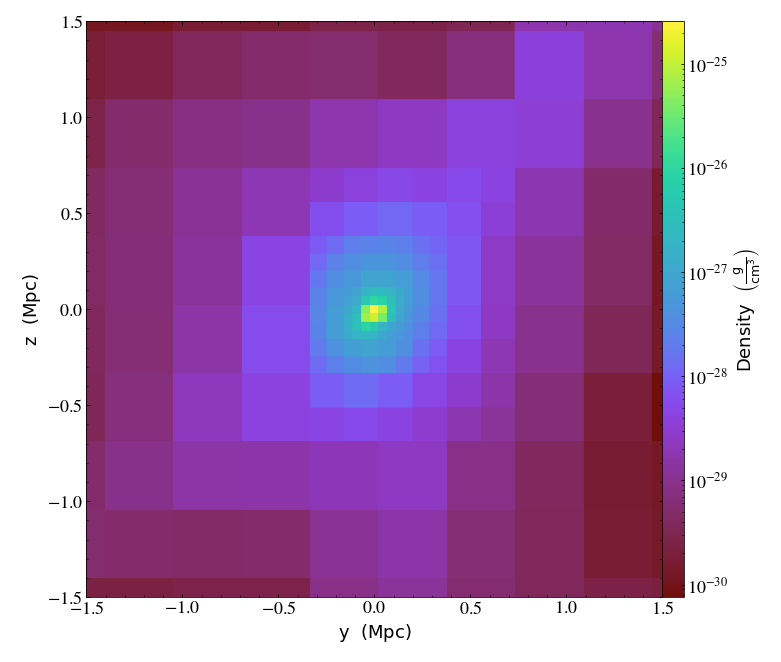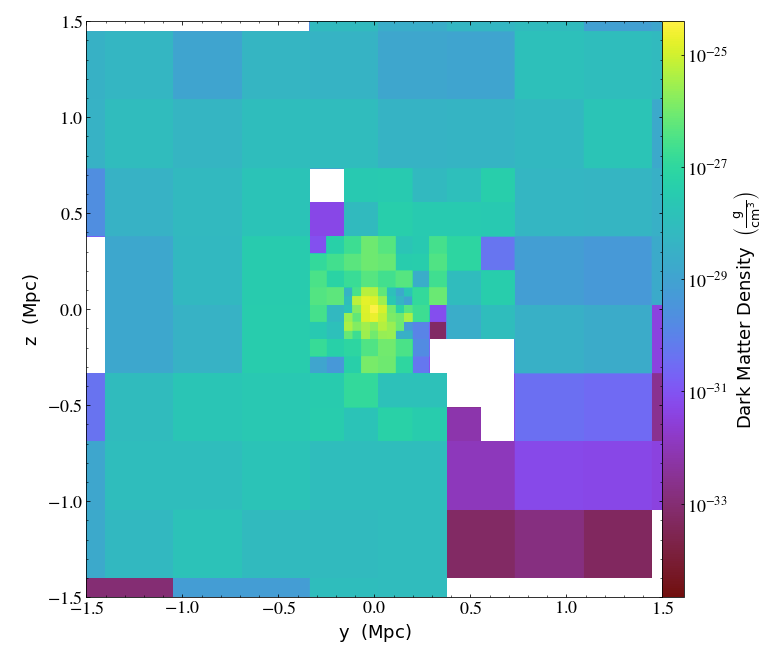# Particle Trajectories¶

Notebook

One can create particle trajectories from a DatasetSeries object for a specified list of particles identified by their unique indices using the particle_trajectories method.

In :
%matplotlib inline
import glob
from os.path import join
import yt
from yt.config import ytcfg
path = ytcfg.get("yt", "test_data_dir")
import matplotlib.pyplot as plt
from mpl_toolkits.mplot3d import Axes3D


First, let's start off with a FLASH dataset containing only two particles in a mutual circular orbit. We can get the list of filenames this way:

In :
my_fns = glob.glob(join(path, "Orbit", "orbit_hdf5_chk_00[0-9][0-9]"))
my_fns.sort()


And let's define a list of fields that we want to include in the trajectories. The position fields will be included by default, so let's just ask for the velocity fields:

In :
fields = ["particle_velocity_x", "particle_velocity_y", "particle_velocity_z"]


There are only two particles, but for consistency's sake let's grab their indices from the dataset itself:

In :
ds = yt.load(my_fns)
dd = ds.all_data()
indices = dd["all", "particle_index"].astype("int")
print (indices)

[1 2] dimensionless


which is what we expected them to be. Now we're ready to create a DatasetSeries object and use it to create particle trajectories:

In :
ts = yt.DatasetSeries(my_fns)
# suppress_logging=True cuts down on a lot of noise
trajs = ts.particle_trajectories(indices, fields=fields, suppress_logging=True)

/tmp/yt/yt/data_objects/data_containers.py:1421: VisibleDeprecationWarning: The requested field name 'particle_velocity_x' is ambiguous and corresponds to any one of the following field types:
{'all', 'nbody'}
Please specify the requested field as an explicit tuple (ftype, fname).
Defaulting to '("all", "particle_velocity_x")'.
Deprecated since v4.0.0. This feature will be removed in v4.1.0
finfo = self.ds._get_field_info(field)
/tmp/yt/yt/data_objects/data_containers.py:1421: VisibleDeprecationWarning: The requested field name 'particle_velocity_y' is ambiguous and corresponds to any one of the following field types:
{'all', 'nbody'}
Please specify the requested field as an explicit tuple (ftype, fname).
Defaulting to '("all", "particle_velocity_y")'.
Deprecated since v4.0.0. This feature will be removed in v4.1.0
finfo = self.ds._get_field_info(field)
/tmp/yt/yt/data_objects/data_containers.py:1421: VisibleDeprecationWarning: The requested field name 'particle_velocity_z' is ambiguous and corresponds to any one of the following field types:
{'all', 'nbody'}
Please specify the requested field as an explicit tuple (ftype, fname).
Defaulting to '("all", "particle_velocity_z")'.
Deprecated since v4.0.0. This feature will be removed in v4.1.0
finfo = self.ds._get_field_info(field)

---------------------------------------------------------------------------
RuntimeError                              Traceback (most recent call last)
/tmp/ipykernel_58846/1430733157.py in <module>
1 ts = yt.DatasetSeries(my_fns)
2 # suppress_logging=True cuts down on a lot of noise
----> 3 trajs = ts.particle_trajectories(indices, fields=fields, suppress_logging=True)

/tmp/yt/yt/data_objects/time_series.py in particle_trajectories(self, indices, fields, suppress_logging, ptype)
480         particle disappear.
481         """
--> 482         return ParticleTrajectories(
483             self, indices, fields=fields, suppress_logging=suppress_logging, ptype=ptype
484         )

/tmp/yt/yt/data_objects/particle_trajectories.py in __init__(self, outputs, indices, fields, suppress_logging, ptype)
146
147         # Instantiate fields the caller requested
--> 148         self._get_data(fields)
149
150     def has_key(self, key):

/tmp/yt/yt/data_objects/particle_trajectories.py in _get_data(self, fields)
277                 # This will fail for non-grid index objects
278                 for grid in particle_grids:
--> 279                     cube = grid.retrieve_ghost_zones(1, grid_fields)
280                     for field in grid_fields:
281                         CICSample_3(

/tmp/yt/yt/data_objects/index_subobjects/grid_patch.py in retrieve_ghost_zones(self, n_zones, fields, all_levels, smoothed)
264             )
265         else:
--> 266             cube = self.ds.covering_grid(
267                 level, new_left_edge, field_parameters=field_parameters, **kwargs
268             )

/tmp/yt/yt/data_objects/construction_data_containers.py in __init__(self, level, left_edge, dims, fields, ds, num_ghost_zones, use_pbar, field_parameters)
653         ).astype("int64")
654         self._setup_data_source()
--> 655         self.get_data(fields)
656
657     def to_xarray(self, fields=None):

/tmp/yt/yt/data_objects/construction_data_containers.py in get_data(self, fields)
827         for a, f in sorted(alias.items()):
828             if f.sampling_type == "particle" and not is_sph_field:
--> 829                 self[a] = self._data_source[f]
830             else:
831                 self[a] = f(self)

/tmp/yt/yt/data_objects/data_containers.py in __getitem__(self, key)
256                 return self.field_data[f]
257             else:
--> 258                 self.get_data(f)
259         # fi.units is the unit expression string. We depend on the registry
260         # hanging off the dataset to define this unit object.

/tmp/yt/yt/data_objects/selection_objects/data_selection_objects.py in get_data(self, fields)
128     def get_data(self, fields=None):
129         if self._current_chunk is None:
--> 130             self.index._identify_base_chunk(self)
131         if fields is None:
132             return

/tmp/yt/yt/geometry/grid_geometry_handler.py in _identify_base_chunk(self, dobj)
333             dobj._chunk_info = weakref.proxy(dobj)
334         elif getattr(dobj, "_grids", None) is None:
--> 335             gi = dobj.selector.select_grids(
336                 self.grid_left_edge, self.grid_right_edge, self.grid_levels
337             )

/tmp/yt/yt/data_objects/selection_objects/data_selection_objects.py in selector(self)
78             )
79         else:
---> 80             self._selector = sclass(self)
81         return self._selector
82

/tmp/yt/yt/geometry/_selection_routines/region_selector.pxi in yt.geometry.selection_routines.RegionSelector.__init__()

RuntimeError: yt attempted to read outside the boundaries of a non-periodic domain along dimension 0.
Region left edge = -0.0625 code_length, Region right edge = 0.5625 code_length
Dataset left edge = 0.0 code_length, Dataset right edge = 1.0 code_length

This commonly happens when trying to compute ghost cells up to the domain boundary. Two possible solutions are to select a smaller region that does not border domain edge (see https://yt-project.org/docs/analyzing/objects.html?highlight=region)
or override the periodicity with
ds.force_periodicity()

The ParticleTrajectories object trajs is essentially a dictionary-like container for the particle fields along the trajectory, and can be accessed as such:

In :
print (trajs["all", "particle_position_x"])
print (trajs["all", "particle_position_x"].shape)

---------------------------------------------------------------------------
NameError                                 Traceback (most recent call last)
/tmp/ipykernel_58846/173114137.py in <module>
----> 1 print (trajs["all", "particle_position_x"])
2 print (trajs["all", "particle_position_x"].shape)

NameError: name 'trajs' is not defined

Note that each field is a 2D NumPy array with the different particle indices along the first dimension and the times along the second dimension. As such, we can access them individually by indexing the field:

In :
plt.figure(figsize=(6, 6))
plt.plot(trajs["all", "particle_position_x"], trajs["all", "particle_position_y"])
plt.plot(trajs["all", "particle_position_x"], trajs["all", "particle_position_y"])

---------------------------------------------------------------------------
NameError                                 Traceback (most recent call last)
/tmp/ipykernel_58846/944358191.py in <module>
1 plt.figure(figsize=(6, 6))
----> 2 plt.plot(trajs["all", "particle_position_x"], trajs["all", "particle_position_y"])
3 plt.plot(trajs["all", "particle_position_x"], trajs["all", "particle_position_y"])

NameError: name 'trajs' is not defined
<Figure size 432x432 with 0 Axes>

And we can plot the velocity fields as well:

In :
plt.figure(figsize=(6, 6))
plt.plot(trajs["all", "particle_velocity_x"], trajs["all", "particle_velocity_y"])
plt.plot(trajs["all", "particle_velocity_x"], trajs["all", "particle_velocity_y"])

---------------------------------------------------------------------------
NameError                                 Traceback (most recent call last)
/tmp/ipykernel_58846/227219977.py in <module>
1 plt.figure(figsize=(6, 6))
----> 2 plt.plot(trajs["all", "particle_velocity_x"], trajs["all", "particle_velocity_y"])
3 plt.plot(trajs["all", "particle_velocity_x"], trajs["all", "particle_velocity_y"])

NameError: name 'trajs' is not defined
<Figure size 432x432 with 0 Axes>

If we want to access the time along the trajectory, we use the key "particle_time":

In :
plt.figure(figsize=(6, 6))
plt.plot(trajs["particle_time"], trajs["particle_velocity_x"])
plt.plot(trajs["particle_time"], trajs["particle_velocity_y"])

---------------------------------------------------------------------------
NameError                                 Traceback (most recent call last)
/tmp/ipykernel_58846/1465126668.py in <module>
1 plt.figure(figsize=(6, 6))
----> 2 plt.plot(trajs["particle_time"], trajs["particle_velocity_x"])
3 plt.plot(trajs["particle_time"], trajs["particle_velocity_y"])

NameError: name 'trajs' is not defined
<Figure size 432x432 with 0 Axes>

Alternatively, if we know the particle index we'd like to examine, we can get an individual trajectory corresponding to that index:

In :
particle1 = trajs.trajectory_from_index(1)
plt.figure(figsize=(6, 6))
plt.plot(particle1["all", "particle_time"], particle1["all", "particle_position_x"])
plt.plot(particle1["all", "particle_time"], particle1["all", "particle_position_y"])

---------------------------------------------------------------------------
NameError                                 Traceback (most recent call last)
/tmp/ipykernel_58846/3293632165.py in <module>
----> 1 particle1 = trajs.trajectory_from_index(1)
2 plt.figure(figsize=(6, 6))
3 plt.plot(particle1["all", "particle_time"], particle1["all", "particle_position_x"])
4 plt.plot(particle1["all", "particle_time"], particle1["all", "particle_position_y"])

NameError: name 'trajs' is not defined

Now let's look at a more complicated (and fun!) example. We'll use an Enzo cosmology dataset. First, we'll find the maximum density in the domain, and obtain the indices of the particles within some radius of the center. First, let's have a look at what we're getting:

In :
ds = yt.load("enzo_tiny_cosmology/DD0046/DD0046")
slc = yt.SlicePlot(ds, "x", [("gas", "density"), ("gas", "dark_matter_density")], center="max", width=(3.0, "Mpc"))
slc.show()So far, so good--it looks like we've centered on a galaxy cluster. Let's grab all of the dark matter particles within a sphere of 0.5 Mpc (identified by "particle_type == 1"):

In :
sp = ds.sphere("max", (0.5, "Mpc"))
indices = sp["all", "particle_index"][sp["all", "particle_type"] == 1]


Next we'll get the list of datasets we want, and create trajectories for these particles:

In :
my_fns = glob.glob(join(path, "enzo_tiny_cosmology/DD*/*.hierarchy"))
my_fns.sort()
ts = yt.DatasetSeries(my_fns)
trajs = ts.particle_trajectories(indices, fields=fields, suppress_logging=True)

/tmp/yt/yt/data_objects/data_containers.py:1421: VisibleDeprecationWarning: The requested field name 'particle_velocity_x' is ambiguous and corresponds to any one of the following field types:
{'all', 'nbody'}
Please specify the requested field as an explicit tuple (ftype, fname).
Defaulting to '("all", "particle_velocity_x")'.
Deprecated since v4.0.0. This feature will be removed in v4.1.0
finfo = self.ds._get_field_info(field)
/tmp/yt/yt/data_objects/data_containers.py:1421: VisibleDeprecationWarning: The requested field name 'particle_velocity_y' is ambiguous and corresponds to any one of the following field types:
{'all', 'nbody'}
Please specify the requested field as an explicit tuple (ftype, fname).
Defaulting to '("all", "particle_velocity_y")'.
Deprecated since v4.0.0. This feature will be removed in v4.1.0
finfo = self.ds._get_field_info(field)
/tmp/yt/yt/data_objects/data_containers.py:1421: VisibleDeprecationWarning: The requested field name 'particle_velocity_z' is ambiguous and corresponds to any one of the following field types:
{'all', 'nbody'}
Please specify the requested field as an explicit tuple (ftype, fname).
Defaulting to '("all", "particle_velocity_z")'.
Deprecated since v4.0.0. This feature will be removed in v4.1.0
finfo = self.ds._get_field_info(field)

---------------------------------------------------------------------------
ValueError                                Traceback (most recent call last)
/tmp/ipykernel_58846/1730623604.py in <module>
2 my_fns.sort()
3 ts = yt.DatasetSeries(my_fns)
----> 4 trajs = ts.particle_trajectories(indices, fields=fields, suppress_logging=True)

/tmp/yt/yt/data_objects/time_series.py in particle_trajectories(self, indices, fields, suppress_logging, ptype)
480         particle disappear.
481         """
--> 482         return ParticleTrajectories(
483             self, indices, fields=fields, suppress_logging=suppress_logging, ptype=ptype
484         )

/tmp/yt/yt/data_objects/particle_trajectories.py in __init__(self, outputs, indices, fields, suppress_logging, ptype)
146
147         # Instantiate fields the caller requested
--> 148         self._get_data(fields)
149
150     def has_key(self, key):

/tmp/yt/yt/data_objects/particle_trajectories.py in _get_data(self, fields)
279                     cube = grid.retrieve_ghost_zones(1, grid_fields)
280                     for field in grid_fields:
--> 281                         CICSample_3(
282                             x,
283                             y,

/tmp/yt/yt/utilities/lib/particle_mesh_operations.pyx in yt.utilities.lib.particle_mesh_operations.CICSample_3()

ValueError: Buffer has wrong number of dimensions (expected 3, got 1)

Matplotlib can make 3D plots, so let's pick three particle trajectories at random and look at them in the volume:

In :
fig = plt.figure(figsize=(8.0, 8.0))
ax.plot(trajs["all", "particle_position_x"], trajs["all", "particle_position_y"], trajs["all", "particle_position_z"])
ax.plot(trajs["all", "particle_position_x"], trajs["all", "particle_position_y"], trajs["all", "particle_position_z"])
ax.plot(trajs["all", "particle_position_x"], trajs["all", "particle_position_y"], trajs["all", "particle_position_z"])

---------------------------------------------------------------------------
NameError                                 Traceback (most recent call last)
/tmp/ipykernel_58846/471695988.py in <module>
1 fig = plt.figure(figsize=(8.0, 8.0))
----> 3 ax.plot(trajs["all", "particle_position_x"], trajs["all", "particle_position_y"], trajs["all", "particle_position_z"])
4 ax.plot(trajs["all", "particle_position_x"], trajs["all", "particle_position_y"], trajs["all", "particle_position_z"])
5 ax.plot(trajs["all", "particle_position_x"], trajs["all", "particle_position_y"], trajs["all", "particle_position_z"])

NameError: name 'trajs' is not definedIt looks like these three different particles fell into the cluster along different filaments. We can also look at their x-positions only as a function of time:

In :
plt.figure(figsize=(6,6))
plt.plot(trajs["all", "particle_time"], trajs["all", "particle_position_x"])
plt.plot(trajs["all", "particle_time"], trajs["all", "particle_position_x"])
plt.plot(trajs["all", "particle_time"], trajs["all", "particle_position_x"])

---------------------------------------------------------------------------
NameError                                 Traceback (most recent call last)
/tmp/ipykernel_58846/1686050241.py in <module>
1 plt.figure(figsize=(6,6))
----> 2 plt.plot(trajs["all", "particle_time"], trajs["all", "particle_position_x"])
3 plt.plot(trajs["all", "particle_time"], trajs["all", "particle_position_x"])
4 plt.plot(trajs["all", "particle_time"], trajs["all", "particle_position_x"])

NameError: name 'trajs' is not defined
<Figure size 432x432 with 0 Axes>

Suppose we wanted to know the gas density along the particle trajectory, but there wasn't a particle field corresponding to that in our dataset. Never fear! If the field exists as a grid field, yt will interpolate this field to the particle positions and add the interpolated field to the trajectory. To add such a field (or any field, including additional particle fields) we can call the add_fields method:

In :
trajs.add_fields([("gas", "density")])

---------------------------------------------------------------------------
NameError                                 Traceback (most recent call last)
/tmp/ipykernel_58846/3340907592.py in <module>

NameError: name 'trajs' is not defined

We also could have included "density" in our original field list. Now, plot up the gas density for each particle as a function of time:

In :
plt.figure(figsize=(6,6))
plt.plot(trajs["all", "particle_time"], trajs["gas", "density"])
plt.plot(trajs["all", "particle_time"], trajs["gas", "density"])
plt.plot(trajs["all", "particle_time"], trajs["gas", "density"])
plt.yscale("log")

---------------------------------------------------------------------------
NameError                                 Traceback (most recent call last)
/tmp/ipykernel_58846/2687267876.py in <module>
1 plt.figure(figsize=(6,6))
----> 2 plt.plot(trajs["all", "particle_time"], trajs["gas", "density"])
3 plt.plot(trajs["all", "particle_time"], trajs["gas", "density"])
4 plt.plot(trajs["all", "particle_time"], trajs["gas", "density"])
5 plt.yscale("log")

NameError: name 'trajs' is not defined
<Figure size 432x432 with 0 Axes>

Finally, the particle trajectories can be written to disk. Two options are provided: ASCII text files with a column for each field and the time, and HDF5 files:

In :
trajs.write_out("halo_trajectories") # This will write a separate file for each trajectory
trajs.write_out_h5("halo_trajectories.h5") # This will write all trajectories to a single file

---------------------------------------------------------------------------
NameError                                 Traceback (most recent call last)
/tmp/ipykernel_58846/2238590062.py in <module>
----> 1 trajs.write_out("halo_trajectories") # This will write a separate file for each trajectory
2 trajs.write_out_h5("halo_trajectories.h5") # This will write all trajectories to a single file

NameError: name 'trajs' is not defined